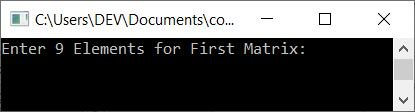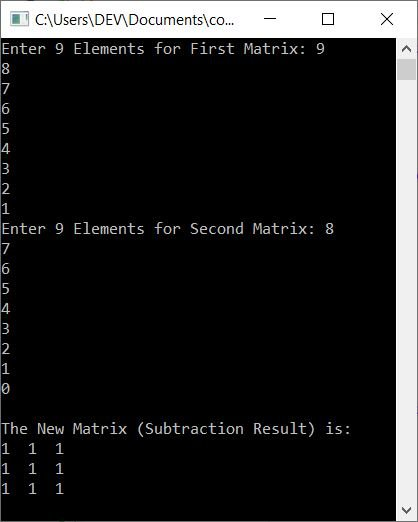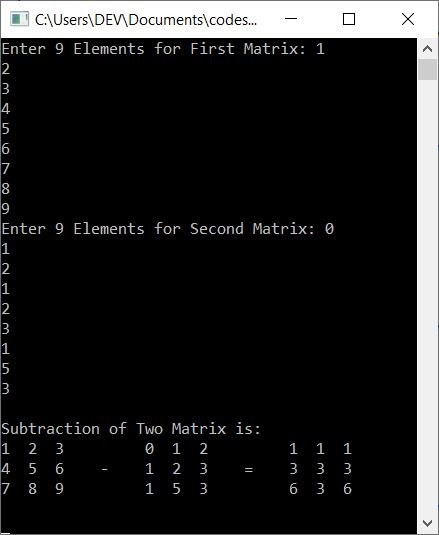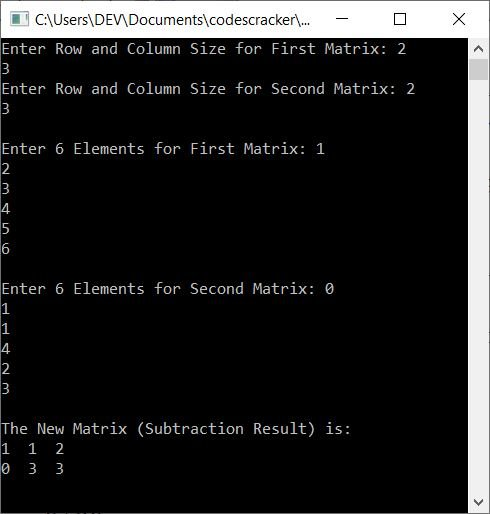# C++ Program to Subtract Two Matrices

In this article, you will learn and get code to subtract two matrices using a C++ program. Here is the list of programs for matrix subtraction:

However, if you are unfamiliar with the topic, you should read the article on matrix subtraction before proceeding with these programs.

Note: In matrix subtraction, the same row and column elements of the second matrix get subtracted from the same row and column elements of the first matrix. Let me give you an example:

```1  2  3     0  1  3     1  1  0
4  5  6  -  3  1  2  =  1  4  4
7  8  9     1  2  3     6  6  6```

As you can see, element (0) of the first row, first column of the second matrix is subtracted from the element (1) of the first row, first column of the first matrix (1). Similarly, element (1) of the second matrix is subtracted from element (2) of the first matrix, and so on.

## Subtract Two 3*3 Matrices in C++

To subtract two matrices in C++ programming, you have to ask the user to enter the two 3*3 matrices. That is, the first matrix has nine elements (numbers) and the second matrix has nine elements. Then subtract the second matrix from the first as shown in the program given below:

```#include<iostream>
using namespace std;
int main()
{
int matOne, matTwo, matSub, i, j;
cout<<"Enter 9 Elements for First Matrix: ";
for(i=0; i<3; i++)
{
for(j=0; j<3; j++)
cin>>matOne[i][j];
}
cout<<"Enter 9 Elements for Second Matrix: ";
for(i=0; i<3; i++)
{
for(j=0; j<3; j++)
cin>>matTwo[i][j];
}
for(i=0; i<3; i++)
{
for(j=0; j<3; j++)
matSub[i][j] = matOne[i][j] - matTwo[i][j];
}
cout<<"\nThe New Matrix (Subtraction Result) is:\n";
for(i=0; i<3; i++)
{
for(j=0; j<3; j++)
cout<<matSub[i][j]<<"  ";
cout<<endl;
}
cout<<endl;
return 0;
}```

This program was built and runs under the Code::Blocks IDE. Here is its sample run:Now supply inputs, say 9, 8, 7, 6, 5, 4, 3, 2, and 1 as 9 elements for the first matrix and 8, 7, 6, 5, 4, 3, 2, 1, and 0 as 9 elements for the second matrix. Here is the sample output after subtracting two given matrices:When the user enters 9, 8, 7, 6, 5, 4, 3, 2, and 1 as the 9 elements for the first matrix, then it gets stored in matOne[][] in this way:

• matOne=9
• matOne=8
• matOne=7
• matOne=6
• matOne=5
• and so on up until
• matOne=1

Whereas the 9 elements, say 8, 7, 6, 5, 4, 3, 2, 1, and 0 get stored in the second matrix, say matTwo[][], in a similar way, that is:

• matTwo=8
• matTwo=7
• matTwo=6
• and so on upto
• matTwo=0

Now the matrix subtraction with the following block of code gets performed:

```for(i=0; i<3; i++)
{
for(j=0; j<3; j++)
matSub[i][j] = matOne[i][j] - matTwo[i][j];
}```

When we evaluate this block of code, then its dry run goes like this:

• matSub = matOne - matTwo
• matSub = matOne - matTwo
• matSub = matOne - matTwo
• matSub = matOne - matTwo
• matSub = matOne - matTwo
• matSub = matOne - matTwo
• matSub = matOne - matTwo
• matSub = matOne - matTwo
• matSub = matOne - matTwo

Now, on putting the number or value for each:

• matSub = 9 - 8
• matSub = 8 - 7
• matSub = 7 - 6
• matSub = 6 - 5
• matSub = 5 - 4
• matSub = 4 - 3
• matSub = 3 - 2
• matSub = 2 - 1
• matSub = 1 - 0

As you can see, the subtraction result is always 1; thus, 1 is stored in every index of matSub[][].

#### Subtract two matrices and print A-B=C

This program is similar to the previous program. But there is an extra feature included in this program. That extra feature is that this program shows the generalized way of doing matrix subtraction with A-B=C. Here, A is the first matrix, B is the second matrix, and C is the subtraction result of both matrices.

```#include<iostream>
using namespace std;
int main()
{
int matOne, matTwo, matSub, i, j;
cout<<"Enter 9 Elements for First Matrix: ";
for(i=0; i<3; i++)
{
for(j=0; j<3; j++)
cin>>matOne[i][j];
}
cout<<"Enter 9 Elements for Second Matrix: ";
for(i=0; i<3; i++)
{
for(j=0; j<3; j++)
cin>>matTwo[i][j];
}
for(i=0; i<3; i++)
{
for(j=0; j<3; j++)
matSub[i][j] = matOne[i][j] - matTwo[i][j];
}
cout<<"\nSubtraction of Two Matrix is:\n";
for(i=0; i<3; i++)
{
for(j=0; j<3; j++)
cout<<matOne[i][j]<<"  ";
if(i==1)
cout<<"  -\t";
else
cout<<"\t";
for(j=0; j<3; j++)
cout<<matTwo[i][j]<<"  ";
if(i==1)
cout<<"  =\t";
else
cout<<"\t";
for(j=0; j<3; j++)
cout<<matSub[i][j]<<"  ";
cout<<endl;
}
cout<<endl;
return 0;
}```

Here is its sample run with user input 1, 2, 3, 4, 5, 6, 7, 8, 9 as first matrix elements, and 0, 1, 2, 1, 2, 3, 1, 5, 3 as second matrix elements:### Subtract two matrices of the given size

This program allows the user to define the size of the matrix before entering the elements. The rest of the program is similar to the first program in this article:

```#include<iostream>
using namespace std;
int main()
{
int rowOne, colOne, rowTwo, colTwo, i, j;
int matOne, matTwo, matSub;
cout<<"Enter Row and Column Size for First Matrix: ";
cin>>rowOne>>colOne;
cout<<"Enter Row and Column Size for Second Matrix: ";
cin>>rowTwo>>colTwo;
if(rowOne==rowTwo && colOne==colTwo)
{
cout<<"\nEnter "<<rowOne*colOne<<" Elements for First Matrix: ";
for(i=0; i<rowOne; i++)
{
for(j=0; j<colOne; j++)
cin>>matOne[i][j];
}
cout<<"\nEnter "<<rowTwo*colTwo<<" Elements for Second Matrix: ";
for(i=0; i<rowTwo; i++)
{
for(j=0; j<colTwo; j++)
cin>>matTwo[i][j];
}
cout<<"\nThe New Matrix (Subtraction Result) is:\n";
for(i=0; i<rowOne; i++)
{
for(j=0; j<colOne; j++)
{
matSub[i][j] = matOne[i][j] - matTwo[i][j];
cout<<matSub[i][j]<<"  ";
}
cout<<endl;
}
}
else
cout<<"\nRow and Column Size of Both Matrix Mismatched!";
cout<<endl;
return 0;
}```

Now supply the following inputs:

• 2 as row size and 3 as column size for the first matrix
• 2 as row size and 3 as column size for the second matrix
• 1, 2, 3, 4, 5, 6 as 6 elements for the first matrix
• 0, 1, 1, 4, 2, 3 as 6 elements for the second matrix

After supplying these inputs, here is the sample output:#### The same program in different languages

C++ Quiz

« Previous Program Next Program »